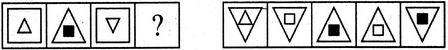Non Verbal Reasoning - Analogy - Discussion

Discussion :: Analogy - Section 1 (Q.No.8)

Each of the following questions consists of two sets of figures. Figures A, B, C and D constitute the Problem Set while figures 1, 2, 3, 4 and 5 constitute the Answer Set. There is a definite relationship between figures A and B. Establish a similar relationship between figures C and D by selecting a suitable figure from the Answer Set that would replace the question mark (?) in fig. (D).

8.

Select a suitable figure from the Answer Figures that would replace the question mark (?).

Problem Figures:                            Answer Figures:(A)     (B)      (C)     (D)                  (1)      (2)      (3)      (4)      (5)

 [A]. 1 [B]. 2 [C]. 3 [D]. 4 [E]. 5

Explanation:

The inner element enlarges to become the outer element while the outer element reduces in size, turns black and becomes the inner element.

 Rohit said: (Sep 6, 2014) But then why triangle is inverted? The Answer could have been C as well! Moreover how is anyone supposed to understand that square has been inverted?

 Rohit said: (Apr 18, 2017) According to me, C is correct answer.

 Bhavna said: (Jun 24, 2018) The answer is C.